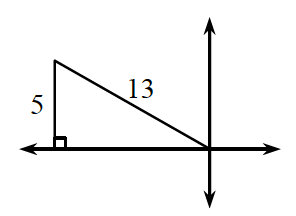### Home > PC > Chapter 4 > Lesson 4.1.3 > Problem4-40

4-40.

If $\text{sin }θ =\frac { 5 } { 13 }$ and $\frac { \pi } { 2 }≤ θ ≤ \pi$ , find $\text{cos }θ$ and $\text{tan }θ$.

Draw and label a right triangle in the correct quadrant.

Calculate the length of the unknown side.
(Or you may know it because it is a Pythagorean Triple!) When you label it, think about if it should be positive or negative.

From the side lengths, you can find the $\text{cos}(θ)$ and the $\text{tan}(θ)$.